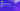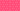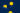Pricing# 7 important financial ratios

Whether you’re looking for ways to grow your business or simply interested in investing, financial ratios are a fundamental component of any analysis. Here’s a breakdown of important financial ratios, and why they’re so useful

## 1. Quick ratio

We’ll start off our list of the most important financial ratios with the quick ratio, also known as the acid test. This is one of the most frequently used types of financial ratios, giving a quick indicator of business liquidity. To calculate the quick ratio, you must subtract current inventory from current assets and then divide this by liabilities:

(Current Assets – Inventory) / Current Liabilities

This shows you how easily a business’s short-term debts will be covered by its existing liquid assets, or cash. If the quick ratio is greater than one, the business is in a good financial position.

## 2. Debt to equity ratio

Another financial ratio to consider is debt to equity. This looks at whether or not a business is borrowing more than it can reasonably pay back using equity as a metric. To calculate debt to equity, you must divide total liabilities by shareholders equity:

Total Liabilities / Shareholders Equity

In other words, this ratio measures the degree to which the business’s operations are funded by debt. When this ratio is greater than one, the company holds more debt. If the value is below one, it indicates that the company holds less debt.

## 3. Working capital ratio

A third ratio pertaining to liabilities is the working capital ratio, also known as the current ratio. Like the quick ratio, this looks at how well a company can pay its existing debts. However, it considers all current assets rather than simply liquid assets, so you don’t need to subtract inventory from the equation. The working capital ratio looks like this when written as a formula:

Current Assets / Current Liabilities

The higher the working capital ratio, the easier it will be for a business to pay off debts using its current assets.

## 4. Price to earnings ratio

We’ve looked at a few of the key financial ratios related to liabilities, but what about those related to earnings? One of the top indicators for earnings potential is the price to earnings ratio, or P/E. This divides a company’s share price by its earnings per share.

Share Price / Earnings per Share

In other words, it measures the amount an investor would pay for each dollar earned. This gives you a quick idea if a stock is under or overvalued. Because share prices vary by industry and market conditions, there isn’t a universal rule for what constitutes a “good” P/E. However, you can compare the company’s P/E to similar stock prices for comparison.

## 5. Earnings per share

Along these same lines is the earnings per share or EPS, another quick ratio to use when assessing future earnings. Earnings per share measures the net income you’ll receive for each share of a company’s stock. To calculate EPS, you must divide net income by the number of outstanding common shares during the financial year.

Net Income / Outstanding Shares

This can potentially be a negative number, if the company has traded at a loss over the year. Usually, investors will look at EPS in combination with a number of other ratios like P/E to determine growth potential.

## 6. Return on equity ratio

Whether you’re investing your own money or interested in keeping shareholders happy, you’ll need to know the return on equity ratio. This is one of the most important financial ratios for calculating profit, looking at a company’s net earnings minus dividends and dividing this figure by shareholders equity.

(Earnings – Dividends) / Shareholders Equity

The result tells you about a company’s overall profitability, and can also be referred to as return on net worth.

## 7. Profit margin

Speaking of profitability, the profit margin is one of the fundamental financial ratios to be aware of. This shows you how efficiently a company is managing its overall costs, or how well it converts revenue into profit. The formula for profit margin is:

Profit / Revenue

You can then multiply the result by 100 to convert it into a percentage. The higher the profit margin, the more efficient the company is in converting sales to profits.

## The bottom line

While these are some of the most important financial ratios, you don’t necessarily need to consider all of them. You can pick and choose the most relevant of these key financial ratios to gain greater understanding of a company’s potential.

## We can help

GoCardless helps you automate payment collection, cutting down on the amount of admin your team needs to deal with when chasing invoices. Find out how GoCardless can help you with ad hoc payments or recurring payments.

Interested in automating the way you get paid? GoCardless can help

Contact sales